Function Repository Resource:

# PeriodicBoxDisplacement

Get the shortest displacement between two points in a box with periodic boundary conditions

Contributed by: Matt Kafker
 ResourceFunction["PeriodicBoxDisplacement"][u,v,L] gives the shortest displacement between vectors u and v in a cubic box of side length L centered on the origin with periodic boundary conditions.

## Details

ResourceFunction["PeriodicBoxDisplacement"] works for boxes in any spatial dimension.

## Examples

### Basic Examples (3)

Compute a 1D periodic box displacement in the "box" represented by the interval [-1,1]:

 In:=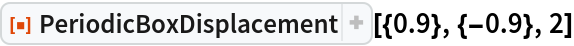Out=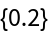PeriodicBoxDisplacement reverts to Euclidean displacement when points are close by within the box:

 In:=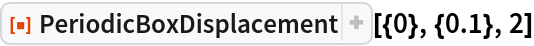Out=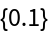Parallel points on the boundaries of the box are mapped to the same point:

 In:=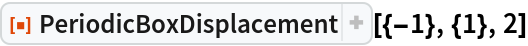Out=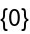### Scope (1)

PeriodicBoxDisplacement also works in higher dimensions:

 In:=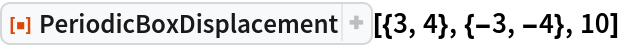Out=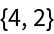### Neat Examples (2)

Visualize the displacement vector between two points in different boxes (dashed boxes are "copies" of the main box due to periodic boundary conditions):

 In:=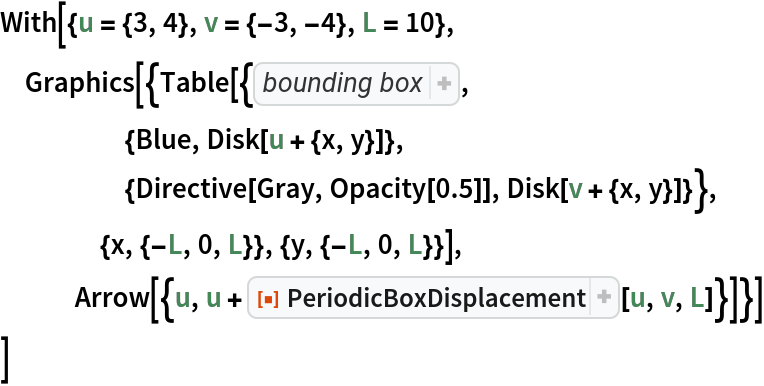Out=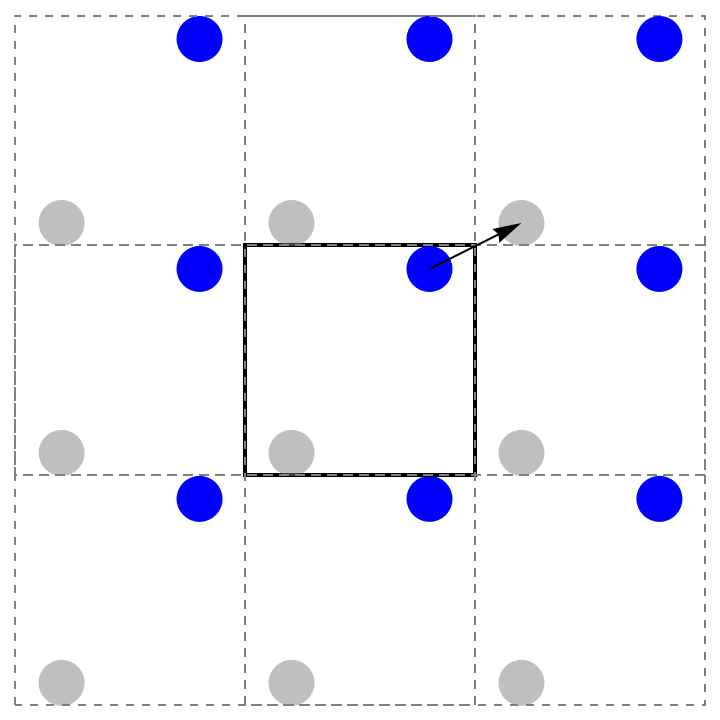In three dimensions:

 In:=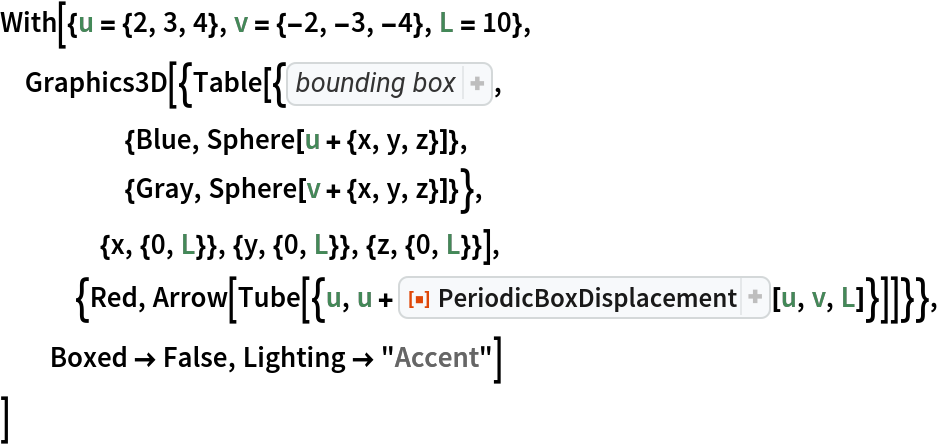Out=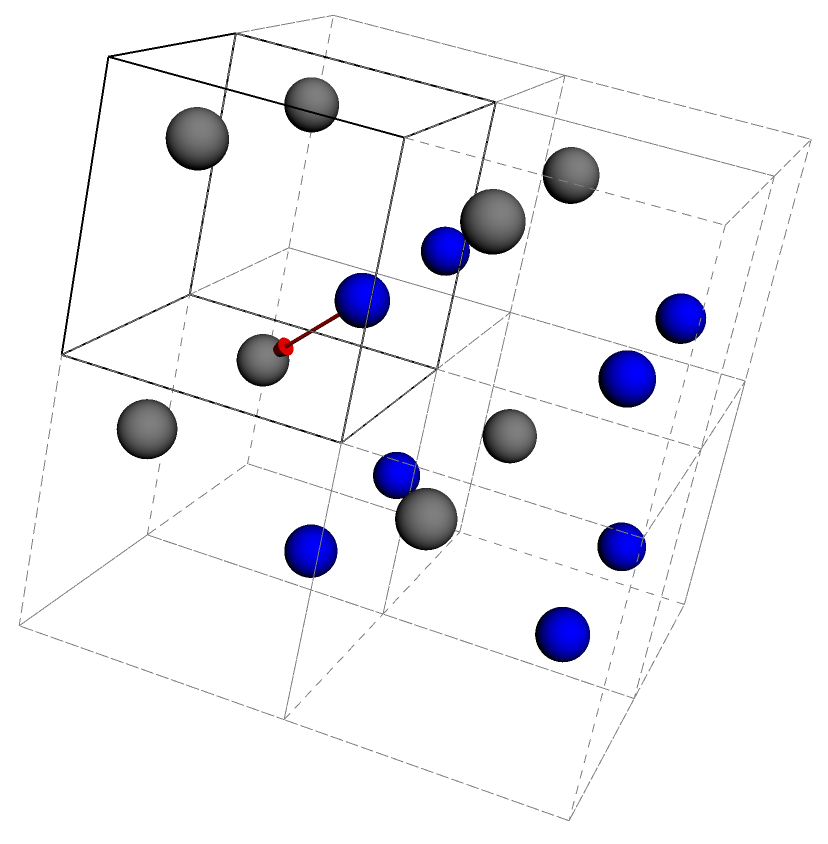## Publisher

Wolfram Summer School

## Version History

• 1.0.0 – 05 August 2021